This project is no longer actively maintained. Please take a look at dm package.

# datamodelr

Define and display data model diagrams:

Use shiny to implement interactive model definition and rendering.

## Installation

```devtools::install_github("bergant/datamodelr")
```

## Usage

### Model Definition in YAML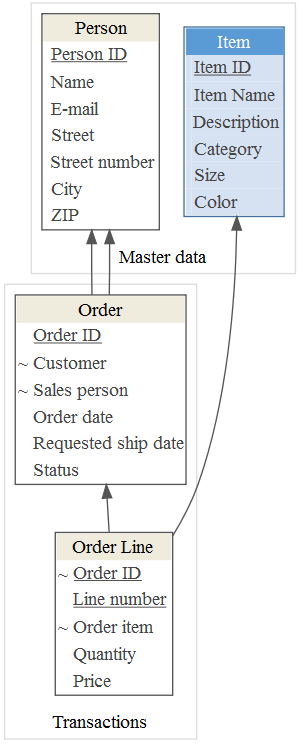Define a data model in YAML:

```# data model segments

- segment: &md Master data
- segment: &tran Transactions

# Tables and columns

- table: Person
segment: *md
columns:
Person ID: {key: yes}
Name:
E-mail:
Street:
Street number:
City:
ZIP:

- table: Order
segment: *tran
columns:
Order ID: {key: yes}
Customer: {ref: Person}
Sales person: {ref: Person}
Order date:
Requested ship date:
Status:

- table: Order Line
segment: *tran
columns:
Order ID: {key: yes, ref: Order}
Line number: {key: yes}
Order item: {ref: Item}
Quantity:
Price:

- table: Item
segment: *md
display: accent1
columns:
Item ID: {key: yes}
Item Name:
Description:
Category:
Size:
Color:
```

Create a data model object with `dm_read_yaml`:

```library(datamodelr)
file_path <- system.file("samples/example.yml", package = "datamodelr")
dm <- dm_read_yaml(file_path)
```

Create a graph object to plot the model:

```graph <- dm_create_graph(dm, rankdir = "BT")
dm_render_graph(graph)
```

### Model Diagram of Interconnected Data Frames

Attach flights database (nycflights13 package) and create a data model from data frames:

```library("nycflights13")
dm_f <- dm_from_data_frames(flights, airlines, weather, airports, planes)
```

Create plot:

```graph <- dm_create_graph(dm_f, rankdir = "BT", col_attr = c("column", "type"))
dm_render_graph(graph)
```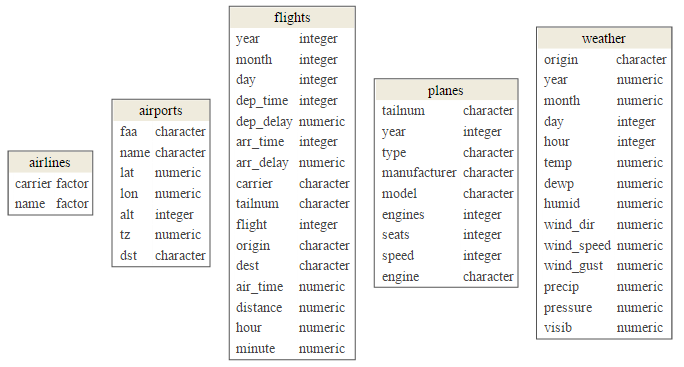Add references and primary keys:

```dm_f <- dm_add_references(
dm_f,

flights\$carrier == airlines\$carrier,
flights\$origin == airports\$faa,
flights\$dest == airports\$faa,
flights\$tailnum == planes\$tailnum,
weather\$origin == airports\$faa
)
graph <- dm_create_graph(dm_f, rankdir = "BT", col_attr = c("column", "type"))
dm_render_graph(graph)
```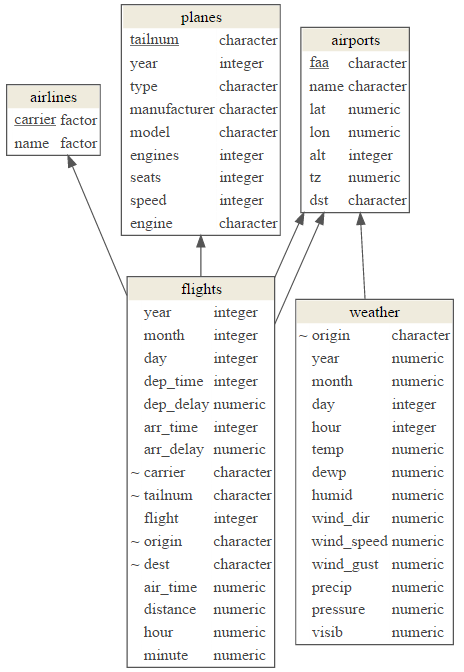### Reverse-engineer SQL Server Database

This example uses Northwind sample database and RODBC package as an interface to SQL Server.

```library(RODBC)
con <- odbcConnect(dsn = "NW")
sQuery <- dm_re_query("sqlserver")
dm_northwind <- sqlQuery(con, sQuery, stringsAsFactors = FALSE, errors=TRUE)
odbcClose(con)

# convert to a data model
dm_northwind <- as.data_model(dm_northwind)
```

Plot the result:

```graph <- dm_create_graph(dm_northwind, rankdir = "BT")
dm_render_graph(graph)
```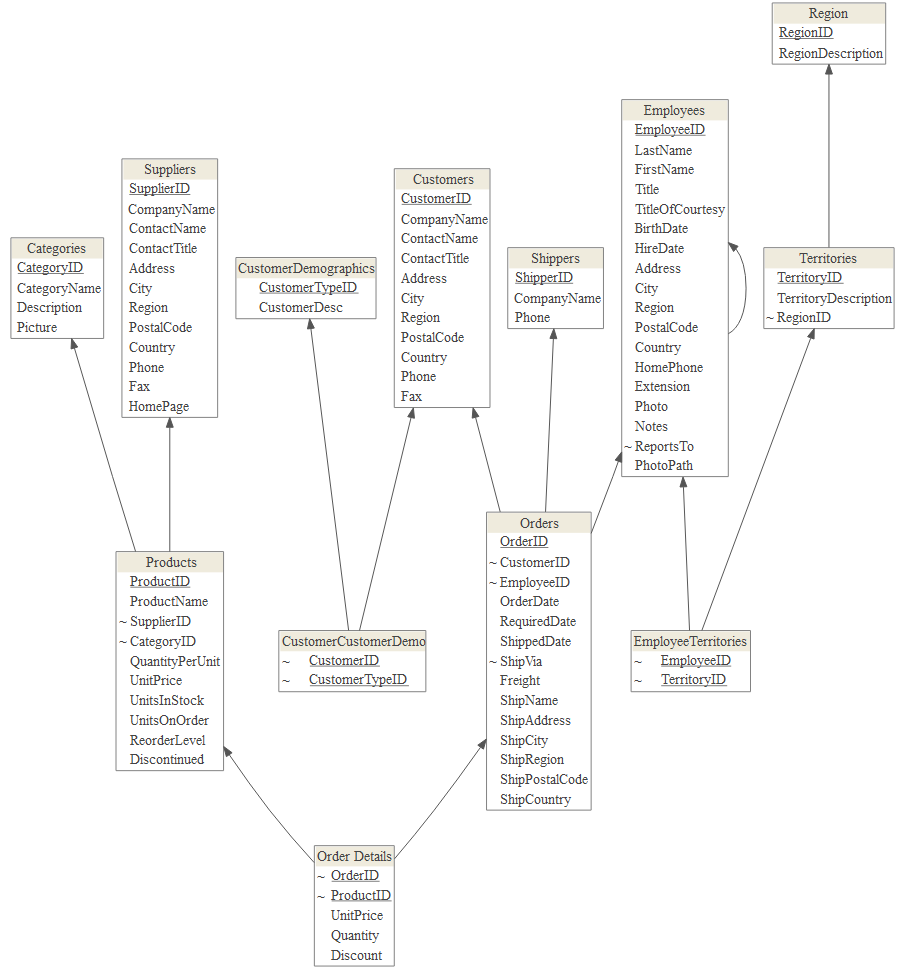### Reverse-engineer PostgreSQL Database

This example uses DVD Rental sample database and RPostgreSQL package as an interface to PostgreSQL database.

```library(RPostgreSQL)
#> Loading required package: DBI
con <- dbConnect(dbDriver("PostgreSQL"), dbname="dvdrental", user ="postgres")
sQuery <- dm_re_query("postgres")
dm_dvdrental <- dbGetQuery(con, sQuery)
dbDisconnect(con)
#>  TRUE

dm_dvdrental <- as.data_model(dm_dvdrental)
```

Show model:

```graph <- dm_create_graph(dm_dvdrental, rankdir = "RL")
dm_render_graph(graph)
```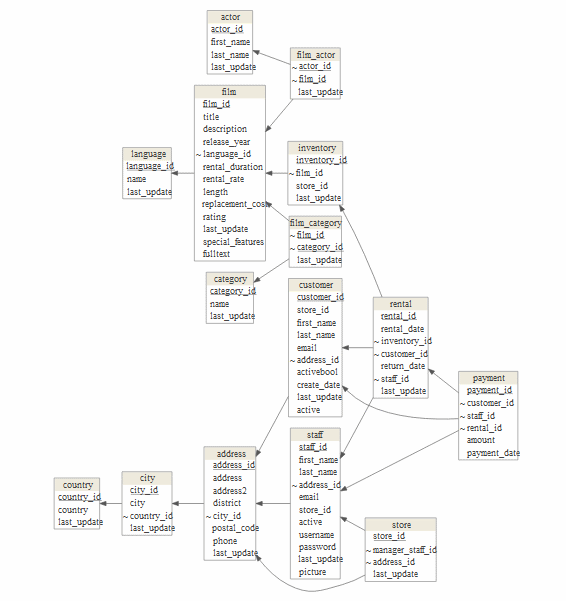### Focused Data Model Diagram

To focus in on a few tables from your model use `focus` attribute in `dm_create_graph` function:

```focus <- list(tables = c(
"customer",
"payment",
"rental",
"inventory",
"film"
))

graph <- dm_create_graph( dm_dvdrental, rankdir = "RL", focus = focus)
dm_render_graph(graph)
```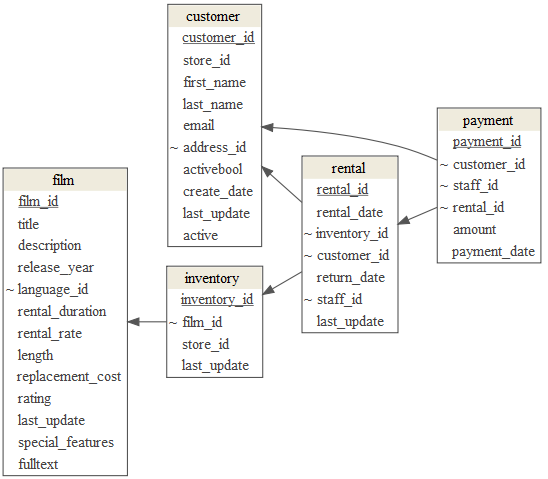### Hide columns

To emphasize table relations and hide the "non-key"" columns use `view_type = "keys_only"`:

```graph <- dm_create_graph(dm_dvdrental, view_type = "keys_only", rankdir = "RL")
dm_render_graph(graph)
```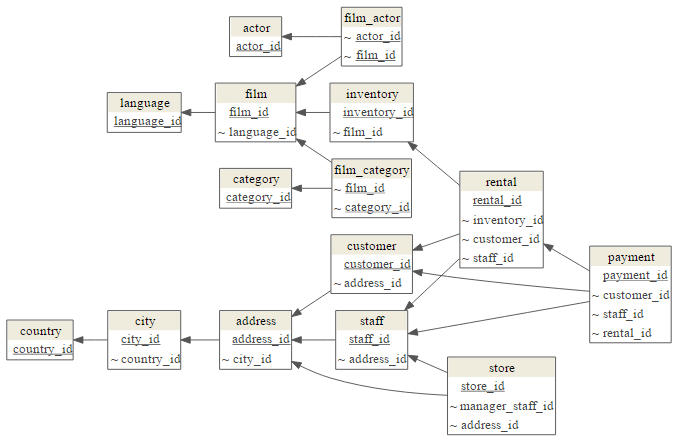### Diagram Segments

Arrange tables in clusters with `dm_set_segment` function:

```table_segments <- list(
Transactions = c("rental", "inventory", "payment"),
Party = c("customer", "staff", "address", "city", "country", "store"),
Film = c("film", "film_actor", "actor", "language", "film_category", "category") )

dm_dvdrental_seg <- dm_set_segment(dm_dvdrental, table_segments)
```

Render diagram with segments:

```graph <- dm_create_graph(dm_dvdrental_seg, rankdir = "RL", view_type = "keys_only")
dm_render_graph(graph)
```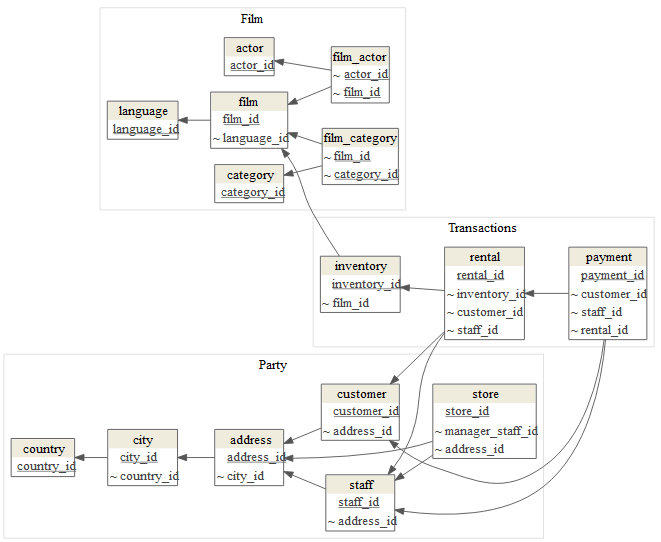### Graph Direction

Use `rankdir` to change the direction of graph:

```graph <- dm_create_graph(dm_dvdrental_seg, rankdir = "BT", view_type = "keys_only")
dm_render_graph(graph)
```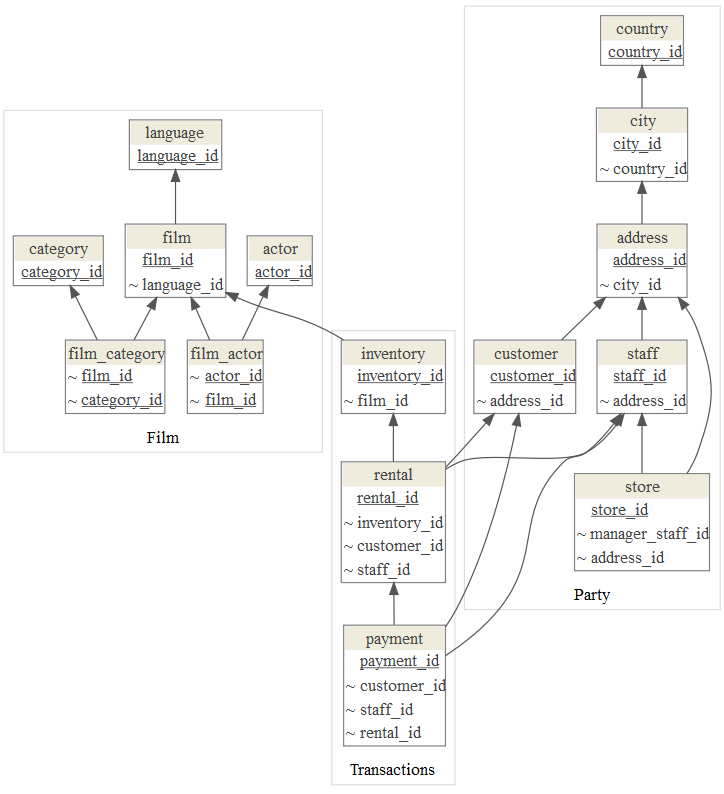### Colors

To emphasise tables with colors use `dm_set_display` function:

```display <- list(
accent1 = c("rental", "payment"),
accent2 = c("customer"),
accent3 = c("staff", "store"),
accent4 = c("film", "actor") )

dm_dvdrental_col <- dm_set_display(dm_dvdrental_seg, display)
graph <- dm_create_graph(dm_dvdrental_col, rankdir = "BT", view_type = "keys_only")
dm_render_graph(graph)
```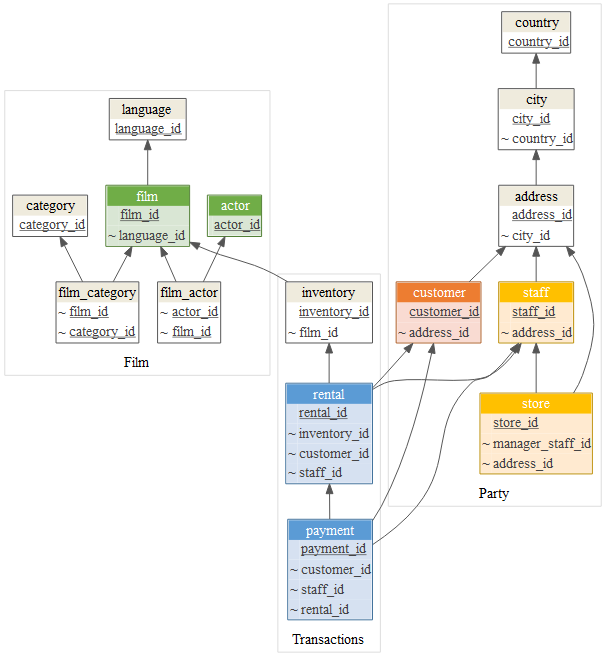Default color scheme includes: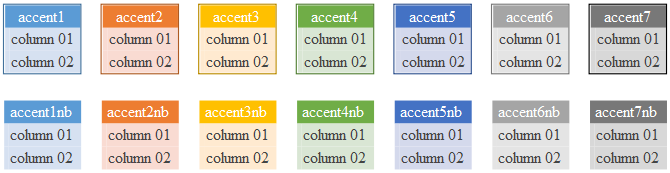### Custom Colors

Add your colors with `dm_add_colors` function:

```my_colors <-
dm_color_scheme(
purple = dm_palette(
line_color = "#8064A2",
header_bgcolor = "#B1A0C7",
header_font = "#FFFFFF",
bgcolor = "#E4DFEC"
),
red = dm_palette(
line_color = "#C0504D",
header_bgcolor = "#DA9694",
header_font = "#FFFFFF",
bgcolor = "#F2DCDB"
)
)

dm_add_colors(my_colors)

dm <- dm_set_display(dm, display = list(
red = c("Order", "Order Line"),
purple = "Item"
))

graph <- dm_create_graph(dm, rankdir = "RL")
dm_render_graph(graph)

```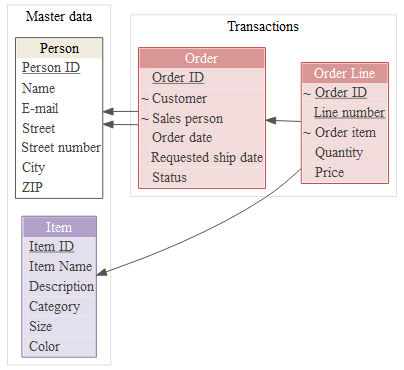### Graphviz Attributes

To change general graph, node or edge graphviz attributes use `graph_attrs`, `edge_attrs` and `node_attrs` arguments when creating graph. This example changes graph background, arrow style (edge attribute) and font (node attribute):

```graph <- dm_create_graph(
dm,
graph_attrs = "rankdir = RL, bgcolor = '#F4F0EF' ",
edge_attrs = "dir = both, arrowtail = crow, arrowhead = odiamond",
node_attrs = "fontname = 'Arial'")

dm_render_graph(graph)
```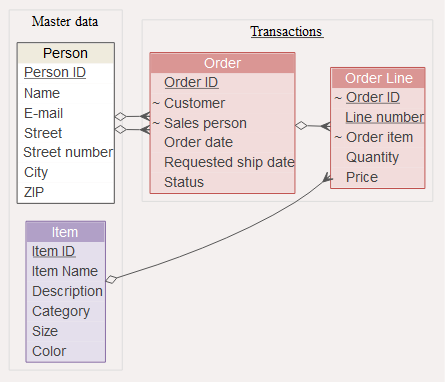### Additional Column Attributes

To include additional column attributes set `col_attr` when creating graph:

```focus <- list(tables = c(
"customer",
"rental",
"inventory",
"film"
))

graph <- dm_create_graph( dm_dvdrental, rankdir = "RL", focus = focus,
col_attr = c("column", "type"))
dm_render_graph(graph)
```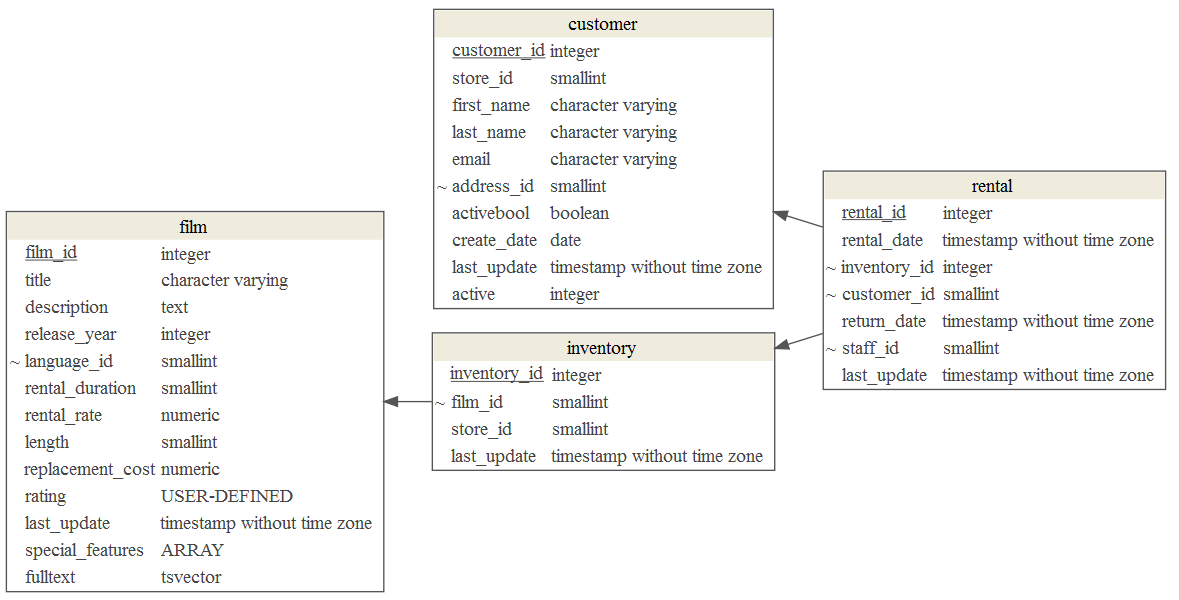### Shiny Application

Try datamodelr Shiny application:

```shiny::runApp(system.file("shiny", package = "datamodelr"))
```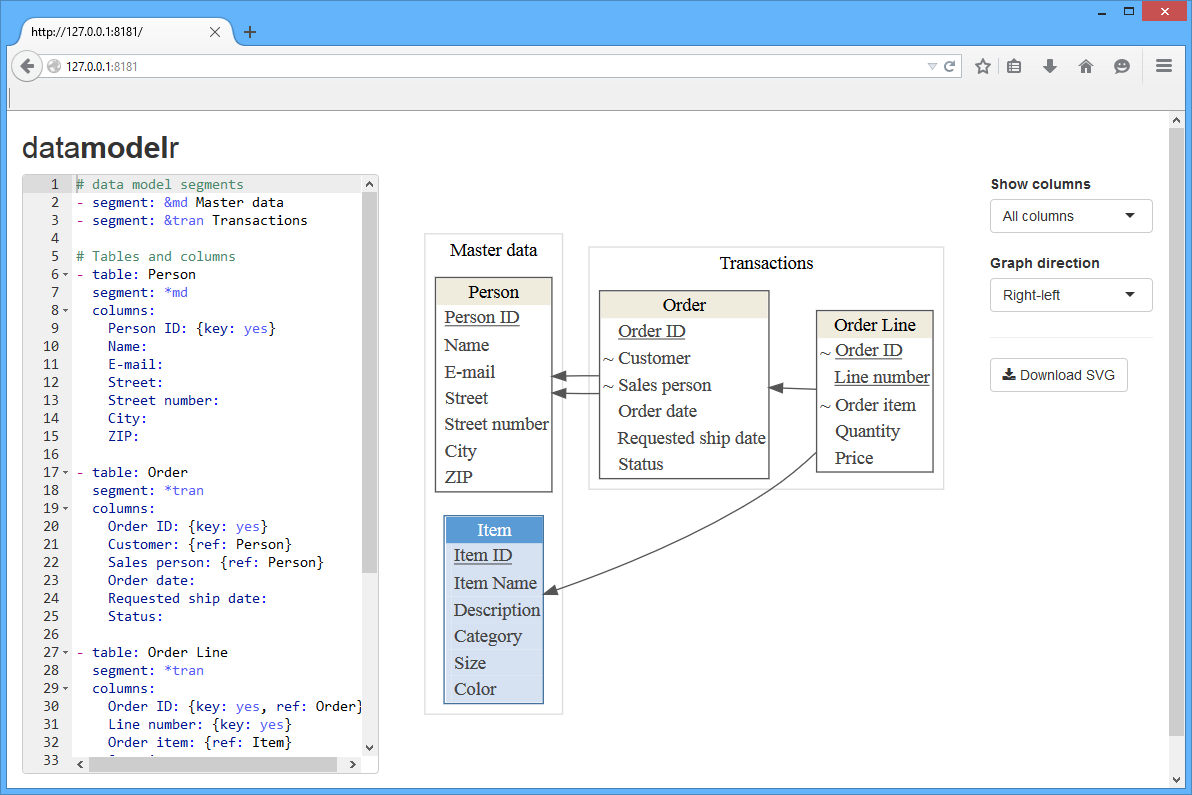## Utilised Packages

datamodelr depends on:

Get A Weekly Email With Trending Projects For These Topics
No Spam. Unsubscribe easily at any time.
r (2,382
data-structures (385